# Shimura-Taniyama conjecture

(diff) ← Older revision | Latest revision (diff) | Newer revision → (diff)

Shimura–Taniyama–Weil conjecture, Taniyama–Shimura conjecture, Taniyama–Weil conjecture, modularity conjecture

A conjecture that postulates a deep connection between elliptic curves (cf. Elliptic curve) over the rational numbers and modular forms (cf. Modular form). It has been completely proved thanks to the fundamental work of A. Wiles and R. Taylor [a5], [a4], and its further refinements [a3], [a2].

Let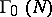be the group of matrices inwhich are upper-triangular modulo a given positive integer. It acts as a discrete group of Mobius transformations (cf. also Discrete group of transformations; Fractional-linear mapping) on the Poincaré upper half-plane(cf. also Poincaré model). A cusp form of weightforis an analytic functiononsatisfying the relation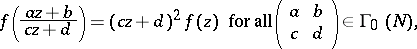together with suitable growth conditions on the boundary of(cf. also Modular form). The functionis periodic of period, and it can be written as a Fourier series in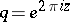with no constant term: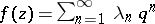. The Dirichlet seriesis called the-function attached to(cf. also Fourier coefficients of automorphic forms; Dirichlet-function). It is essentially the Mellin transform of:The space of cusp forms of weightonis a finite-dimensional vector space and is preserved by the involution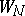defined by. E. Hecke has shown that iflies in one of the two eigenspaces for this involution (with eigenvalue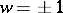), then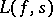satisfies the functional equation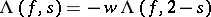, and thathas an analytic continuation to all of.

Letbe an elliptic curve over the rational numbers, and let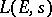denote its Hasse–Weil-series. The curveis said to be modular if there exists a cusp formof weighton, for some, such that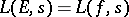. The Shimura–Taniyama conjecture asserts that every elliptic curve overis modular. Thus, it gives a framework for proving the analytic continuation and functional equation for. It is prototypical of a general relationship between the-functions attached to arithmetic objects and those attached to automorphic forms (cf. also Automorphic form), as described in the far-reaching Langlands program.

A. Weil's refinement of the conjecture predicts that the integeris equal to the arithmetic conductor of(cf. also Elliptic curve). One now knows that every elliptic curve is modular. Wiles' method proceeds by viewing the Shimura–Taniyama conjecture in a wider framework which predicts the modularity of the (two-dimensional) Galois representations arising from the cohomology of varieties over(cf. also Galois theory).

The modularity ofcan also be formulated as the statement thatis a quotient of the modular curve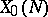over; this curve represents the solution to the moduli problem of classifying pairs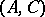consisting of an elliptic curvewith a distinguished cyclic subgroupof order. Alternately, ifis modular, then there is a (non-constant) complex-analytic uniformization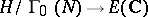.

The importance of the Shimura–Taniyama conjecture is manifold. Firstly, it gives the analytic continuation offor a large class of elliptic curves. The-function itself plays a key role in the study of, most notably through the celebrated Birch–Swinnerton Dyer conjecture. Secondly, the modular curveis endowed with a natural collection of algebraic points arising from the theory of complex multiplication (cf. also Elliptic curve), and the existence of a modular parametrization allows the construction of points ondefined over Abelian extensions of certain imaginary quadratic fields. This fact was exploited by B.H.. Gross and D. Zagier and by V.A. Kolyvagin to give strong evidence for the Birch–Swinnerton Dyer conjecture for, under the assumption thatis modular.

The Shimura–Taniyama conjecture admits various generalizations. Replacingby an arbitrary number field, it predicts that an elliptic curveoveris associated to an automorphic form on. Whenis totally real, such anis often uniformized by a Shimura curve attached to a suitable quaternion algebra (cf. also Quaternion) overwith exactly one split place at infinity (whenis of odd degree, or whenhas at least one prime of multiplicative reduction). In the context of function fields over finite fields, the Shimura–Taniyama conjecture admits an analogue which was established earlier by V.G. Drinfel'd using methods different from those of Wiles.

How to Cite This Entry:
Shimura-Taniyama conjecture. Encyclopedia of Mathematics. URL: http://encyclopediaofmath.org/index.php?title=Shimura-Taniyama_conjecture&oldid=13483
This article was adapted from an original article by H. Darmon (originator), which appeared in Encyclopedia of Mathematics - ISBN 1402006098. See original article